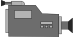Factoring Quadratics (Using Diamond Problems)Factoring Quadratics (Without Using Diamond Problems)1. Factoring Trinomials (Using Diamond Problems):

• Setup:
• Record information:
• Use the information for factoring.

2. Factoring Trinomials (Without Using Diamond Problems):

• Set up:
• Recording the sum:
• Figuring out the factors: